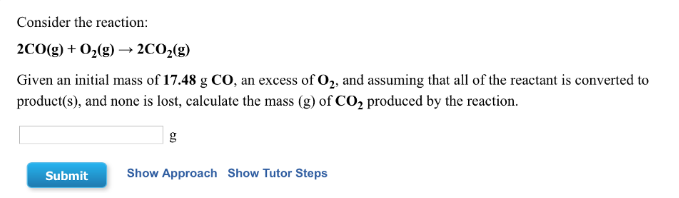# Problem: Consider the reaction: 2CO (g) + O2 (g) → 2CO2 (g) Given an initial mass of 17.48 g CO, an excess of O2, and assuming that all of the reactant is converted to product(s), and none is lost, calculate the mass (g) of CO2 produced by the reaction.

###### FREE Expert Solution
82% (495 ratings)###### Problem Details

Consider the reaction:

2CO (g) + O2 (g) → 2CO2 (g)

Given an initial mass of 17.48 g CO, an excess of O2, and assuming that all of the reactant is converted to product(s), and none is lost, calculate the mass (g) of CO2 produced by the reaction.